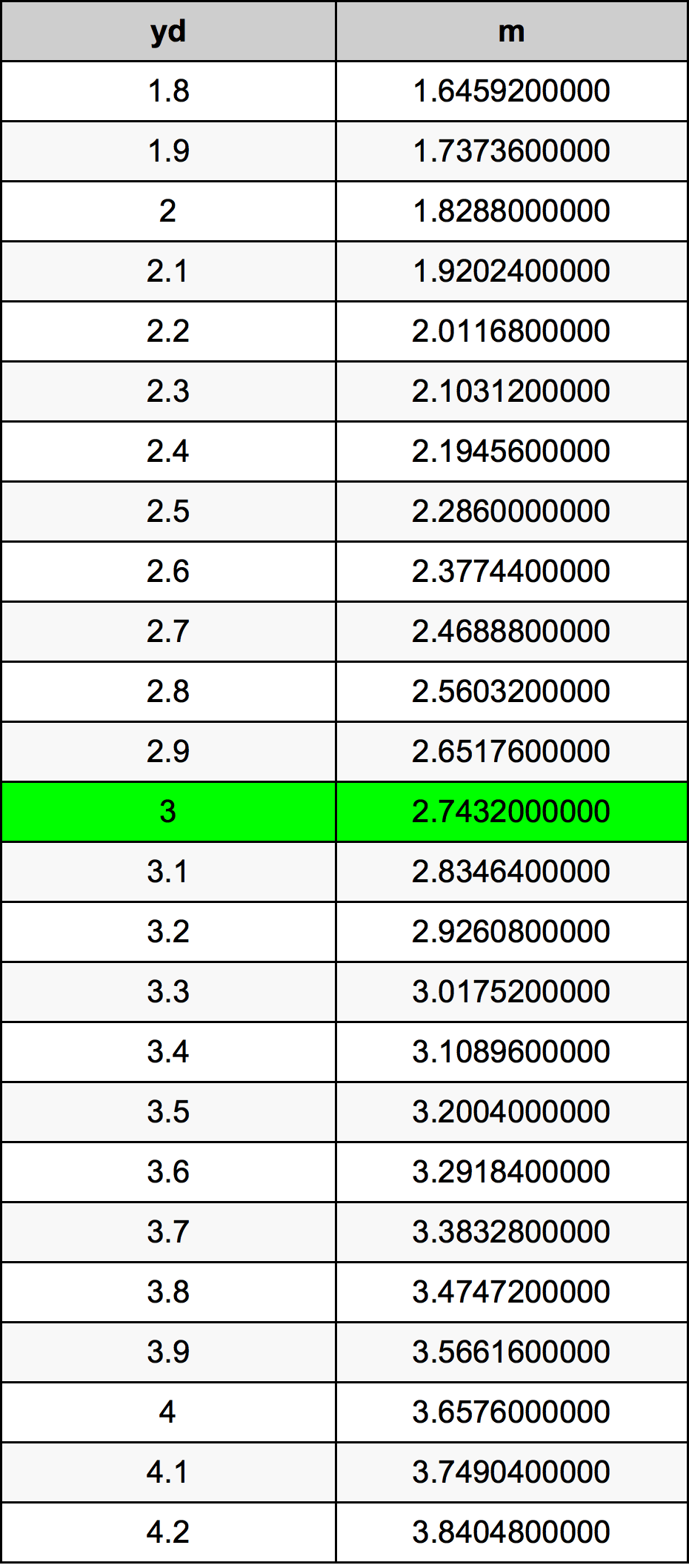Yards To Meters

# 3 yd to m3 Yards to Meters

yd
=
m

## How to convert 3 yards to meters?

 3 yd * 0.9144 m = 2.7432 m 1 yd
A common question is How many yard in 3 meter? And the answer is 3.280839895 yd in 3 m. Likewise the question how many meter in 3 yard has the answer of 2.7432 m in 3 yd.

## How much are 3 yards in meters?

3 yards equal 2.7432 meters (3yd = 2.7432m). Converting 3 yd to m is easy. Simply use our calculator above, or apply the formula to change the length 3 yd to m.

## Convert 3 yd to common lengths

UnitLength
Nanometer2743200000.0 nm
Micrometer2743200.0 µm
Millimeter2743.2 mm
Centimeter274.32 cm
Inch108.0 in
Foot9.0 ft
Yard3.0 yd
Meter2.7432 m
Kilometer0.0027432 km
Mile0.0017045455 mi
Nautical mile0.0014812095 nmi

## What is 3 yards in m?

To convert 3 yd to m multiply the length in yards by 0.9144. The 3 yd in m formula is [m] = 3 * 0.9144. Thus, for 3 yards in meter we get 2.7432 m.

## 3 Yard Conversion Table## Alternative spelling

3 Yard to m, 3 Yard in m, 3 Yards to Meters, 3 Yards in Meters, 3 yd to m, 3 yd in m, 3 Yards to Meter, 3 Yards in Meter, 3 Yards to m, 3 Yards in m, 3 yd to Meters, 3 yd in Meters, 3 yd to Meter, 3 yd in Meter